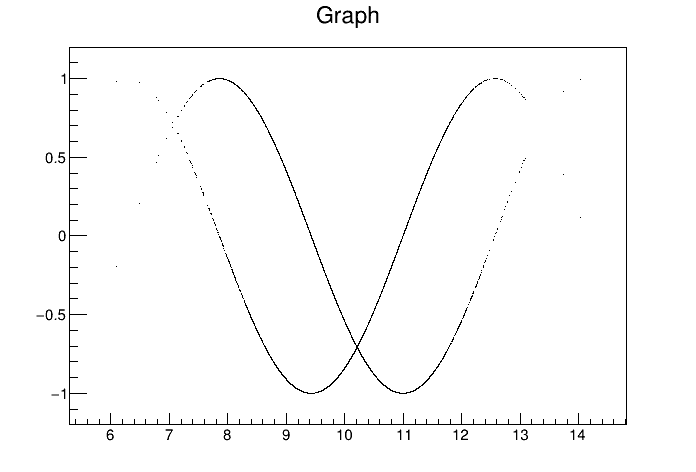# treegetval¶

Illustrates how to retrieve TTree variables in arrays.

This example:

• creates a simple TTree,
• generates TTree variables thanks to the Draw method with goff option,
• retrieves some of them in arrays thanks to GetVal,
• generates and draw graphs with these arrays.

The option goff in TTree::Draw behaves like any other drawing option except that, at the end, no graphics is produced ( goff= graphics off). This allows to generate as many TTree variables as needed. All the graphics options (except para and candle) are limited to four variables only. And para and candle need at least two variables.

Note that by default TTree::Draw creates the arrays obtained with GetVal with a length corresponding to the parameter fEstimate. By default fEstimate=1000000 and can be modified via TTree::SetEstimate. To keep in memory all the results use:

tree->SetEstimate(-1);


SetEstimate should be called if the expected number of selected rows is greater than 1000000.

Author: Olivier Couet
This notebook tutorial was automatically generated with ROOTBOOK-izer from the macro found in the ROOT repository on Thursday, August 18, 2022 at 09:51 AM.

create a simple TTree with 5 branches

In :
Int_t run, evt;
Float_t x,y,z;
TTree *T = new TTree("T","test friend trees");
T->Branch("Run",&run,"Run/I");
T->Branch("Event",&evt,"Event/I");
T->Branch("x",&x,"x/F");
T->Branch("y",&y,"y/F");
T->Branch("z",&z,"z/F");
TRandom r;
for (Int_t i=0;i<10000;i++) {
if (i < 5000) run = 1;
else          run = 2;
evt = i;
x = r.Gaus(10,1);
y = r.Gaus(20,2);
z = r.Landau(2,1);
T->Fill();
}


Draw with option goff and generate seven variables

In :
Int_t n = T->Draw("x:y:z:Run:Event:sin(x):cos(x)","Run==1","goff");
printf("The arrays' dimension is %d\n",n);

The arrays' dimension is 5000


Retrieve variables 0, 5 et 6

In :
Double_t *vx  = T->GetVal(0);
Double_t *vxs = T->GetVal(5);
Double_t *vxc = T->GetVal(6);


Create and draw graphs

In :
TGraph *gs = new TGraph(n,vx,vxs);
TGraph *gc = new TGraph(n,vx,vxc);
gs->Draw("ap");
gc->Draw("p");

Info in <TCanvas::MakeDefCanvas>:  created default TCanvas with name c1


Draw all canvases

In :
gROOT->GetListOfCanvases()->Draw()